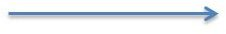# Finding the Measurement of an Angle Using a Protractor: A 4th Grade Lesson

Page content

Identify the measurement of angles using a protractor. Using a protractor, draw angles with a specified measurement. Download a copy of the lesson at the end of the article.

Lesson Objective: The lesson is aligned to the Common Core State Standards for Mathematics – 4MD.6 Geometric Measurement – Measure angles in whole-number degrees using a protractor. Sketch angles of specified measure.

Materials Required: Protractor, ruler

## Lesson Procedure:

Exploring a Protractor

Look at the protractor shown below.

This is a mathematical tool that measures angles in degrees. You can also use the protractor and a ruler to draw angles.

Protractors usually have two sets of numbers. One set of numbers starts at the right. As you read the numbers from right to left, the numbers increase. The other set of numbers starts at the left and increases towards the right. These numbers represent degrees. It is important to use one set of numbers.

Notice at the bottom center of the protractor there is a dot.

## Drawing an Angle using a Protractor and a RulerSteps:

1. Draw a ray using the ruler.
2. Place the dot at the center of the protractor on the endpoint of the ray.
3. Make sure the marking on the protractor that represents 0 degree is on the ray.

4. Find the marking on the protractor that represents 40 degrees.  Place a dot on your paper next to the marking that represents 40 degrees.
5. Remove the protractor. Use the ruler to draw a ray from the endpoint of the first ray to the dot that represents 40 degrees.

## Individual or Group Work:

Use the protractor and ruler to draw angles measuring the following degrees.

1. 35 degrees
2. 75 degrees
3. 110 degrees
4. 142 degrees
5. 156 degrees

Answers:

1. Students draw an angle that measures approximately 35 degrees.
2. Students draw an angle that measures approximately 75 degrees.
3. Students draw an angle that measures approximately 110 degrees.
4. Students draw an angle that measures approximately 142 degrees.
5. Students draw an angle that measures approximately 156 degrees.

Identifying Measurement of an Angle using a Protractor

Steps:

1. Place the dot of the protractor at the vertex of the angle.
2. Place the edge of the protractor on one ray of the angle.
3. Notice where the other ray of the angle lies on the protractor. Look at the degrees on the protractor where the other ray of the angle lies.
4. The angle below measures approximately 19 degrees.

## Individual or Group Work:

Use the protractor to find the following angles measurements in degrees.

## Answers:

1. Angle measures approximately 55 degrees.
2. Angle measures measures approximately 65 degrees.
3. Angle measures measures approximately 132 degrees.
4. Angle measures measures approximately 24 degrees.
5. Angle measures measures approximately 72 degrees.

Students should be able to draw and measure angles with a specified measurement using a protractor and a ruler.

Download a copy of the lesson and group work.

## This post is part of the series: Finding the Measurement of Angles: 4th Grade Lessons

These three lessons will have students learn to find the measurement of angles by using protractors, addition and subtraction. These math lessons all meet Common Core objectives.